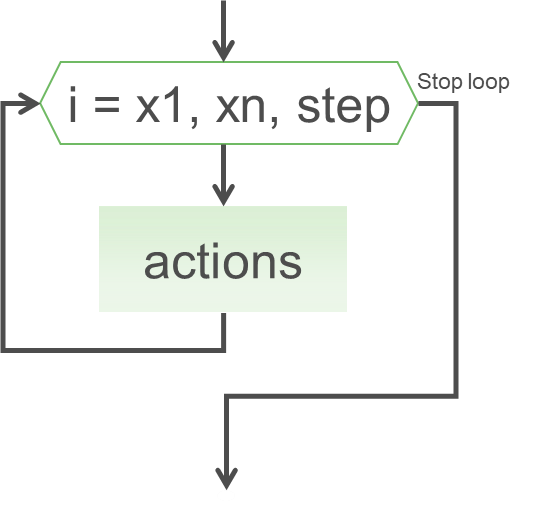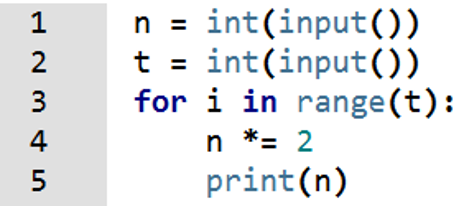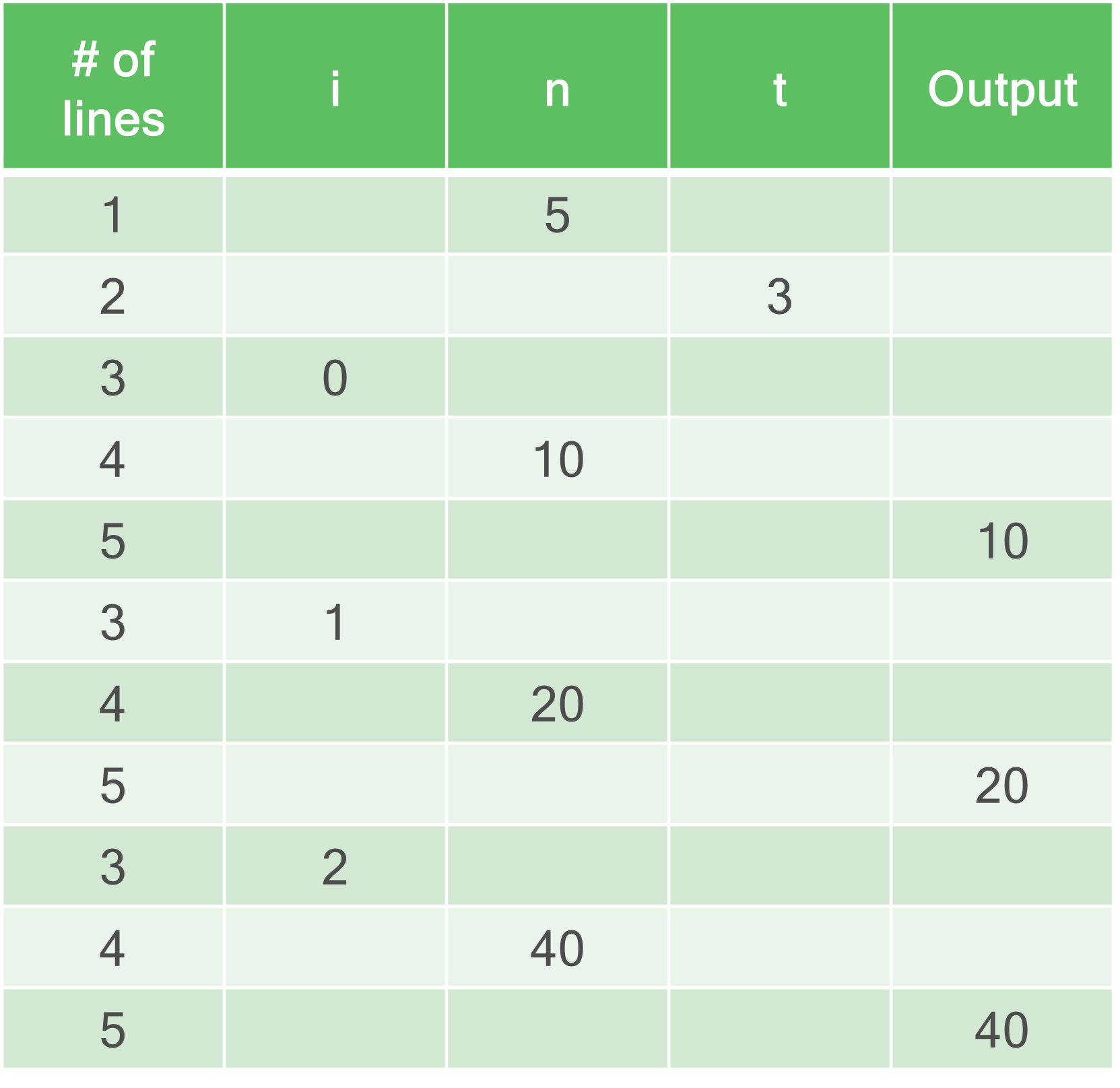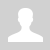Python. FOR loop

11.1.2.4 write program code using a for loop
11.1.2.5 define a range of values for a loop

Python. FOR loop

The for loop executes a block of code a specified number of times.Syntax
```for ... in range(...):      action1                    action2     ...      actionN ```

Like the while loop, the for loop has a header that ends with a colon and the loop's body, which is indented four spaces. In a loop of the form for ... in range(...): instead of the first dot indicates some kind of variable iterator (option) cycle, which will vary and depends on the value range of values (range).

Task 1. Write a program that displays in a row (on separate lines) is an integer from 0 (inclusive) to n (not inclusive).

```n = int(input())  for i in range(n):  # range(n) consists the range from 0 to n-1      print(i)  ```

Task 2. The following program counts the sum of all integers that are less than n.

```n = int(input())  total = 0  for i in range(n):      print("The counter’s value", i)      total += i      print("Subtotal", total)  print("The total of all numbers:", total) ```

Range

The range() function returns a sequence of numbers, starting from 0 by default, and increments by 1 (by default), and stops before a specified number.

If the range is set to one number, the iterator goes from 0 to the preset value (not including).

`range(5) -> [0,1,2,3,4]`

If you specify two numbers, it is the initial value of the iterator and end.

`range(2, 5) -> [2,3,4]`

If there are three numbers, it is not only the start and the end value of the iterator but the step iterator.

`range(0, 5, 2) -> [0, 2,4]`

When to use FOR loop

A for loop is used when some piece of code to be run multiple times, it is known how many times before the start of the loop.

The bacterium divides into two within 1 minute. At the beginning, there are n of bacteria. Write a program for calculating the number of bacteria after t minutes.

Input: Two integers n and t
Output: The number of bacteria after t minutes.

 Code Trace tableQuestions:

Exercises:

Категория: Algorithms | Добавил: bzfar77 (07.10.2021)
Просмотров: 259 | Теги: for loop, Range, iterator, Python | Рейтинг: 0.0/0
 Всего комментариев: 0